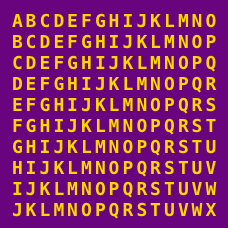Computer Science

# Prime testing

True or False

$98402937$ is a prime number?

Statement

If an odd integer $n>1$ satisfies ${ 2 }^{ n-1 }\equiv 1(mod\quad n)$, then $n$ must be a prime?

What is the smallest number that doesn't satisfy the above statement?

When $n = 3$, $2^{n}-3 = 5$. This is the first value of $n$ for which $2^n - 3$ is prime. What is the $24$th value of $n$ such that $2^{n}-3$ is a prime number?

×